SYLLABUS  Previous: 3.3.2 Itô lemma  Up: 3.3 Improved model using  Next: 3.4 Hedging an option

3.3.3 Evaluate an expectancy or eliminate the uncertainty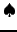[ SLIDE portfolio - no random - no arbitrage - Black-Scholes - properties || VIDEO modem - LAN - DSL]

The Itô lemma shows how it is possible to superpose infinitesimal increments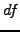to mimic the evolution of the value of a financial derivative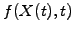, which is a known function of the stochastic spot price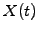. Starting from an initial (alt. terminal) value that is known at a time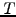, a finite number of incremental changes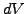can in be accumulated to approximate a single possible outcome at a later (alt. earlier) time: the implementation of the so-called Monte-Carlo method will discussed later with a practical example (sect.4.5). At the end, the fair price for the derivative is calculated as the expectancy from a large number of possible outcomes, i.e. by performing a statistical average where each payoff is properly weighted with the number of times this value has been reached.
The main drawback of a statistical method is the slow convergence (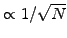) with the number of samples. The problem can be traced back to the difficulty of integrating the stochastic term in the Itô differential (3.3.2#eq.2). By combining anti-correlated assets, it is however possible to reduce the amount of fluctuations in a portfolio. Sometimes, it is even possible to completely eliminate the uncertainty through delta-hedging, in effect transforming the stochastic differential equation (SDE) into a partial differential equation (PDE) that is much simpler to solve. For that

1. Create a portfolio, combining one derivative (e.g. an option) of valuewith a yet unspecified, but constant number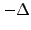of the underlying asset. The initial value of this portfolio and its incremental change per time-step are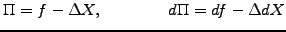(3.3.3#eq.1)

where the Itô differential (3.3.2#eq.2) can be used to substituteand the stochastic differential (3.3.1#eq.1) for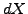.
2. Choose the right amount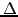of the underlying so as to exactly cancel the random component, which is proportional to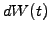in the Itô differential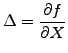(3.3.3#eq.2)

With this choice, the total value of the portfolio becomes deterministic, i.e. the remaining equation has no term left in.
3. No-arbitrage arguments show that without taking any risk, the portfolio has to earn the same as the risk-free interest rate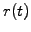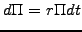(3.3.3#eq.3)

Indeed, if this was not the case and the earnings were larger (alt. smaller), arbitrageurs would immediately borrow money from (alt. lend money to) the market until the derivative expires and make a risk less profit from the difference in the returns.
4. Reassemble the small deterministic incremental values into a partial differential equation, which can be solved more efficiently to obtain the present value of the derivative.
Of course, the amountwill change after a short time and the portfolio has to be continuously re-hedged to obtain a meaningful value for the derivative-which is not quite possible in the real world. Two examples illustrate the procedure in the coming sections, using delta-hedging to calculate the price of derivatives in the stock and the bond markets.

SYLLABUS  Previous: 3.3.2 Itô lemma  Up: 3.3 Improved model using  Next: 3.4 Hedging an option